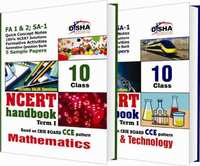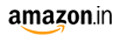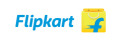Share

# NCERT Handbook Term 1 - Science & Mathematics: Class 10 (NCERT Solutions + FA activities + SA Practice Questions & 5 Sample Papers) CBSE Class 10

SubjectNCERT Handbook Term 1 - Science & Mathematics: Class 10 (NCERT Solutions + FA activities + SA Practice Questions & 5 Sample Papers)By Disha Experts (Author)
M.R.P 400/-275.00(31%)280.00(30%)
Product Description

The set of 2 books “NCERT Handbook Term 1 Science & Mathematics Class 10 (NCERT Solutions + FA activities + SA Practice Questions & 5 Sample Papers)” is exclusively written for CBSE students of class 10. The books provide Quick Revision/Notes of the concepts involved along with important formulas and definitions, in each chapter, which would act as a refresher. This is followed by the detailed solutions (Question-by-Question) of all the questions/exercises provided in the NCERT book for the current session. All the in-chapter questions of the NCERT books, wherever found, have been fully solved. The solutions have been designed in such a manner (Step-by-Step) that it would bring 100% Concept Clarity for the students. The solutions are Complete (each and every question is solved), Inflow (exactly on the flow of questions in the NCERT book) and Correct (Errorless). The book is compliant with the CCE pattern and provides two variety of exercises, Formative and Summative Assessment. The Formative Assessment (for FA1 and FA2) contains exercises based on, Fill in the Blanks, True/False, Matchings, MCQs, passage based questions, activity, crossword, etc. The Summative Assessment (for SA1) contains exercises on MCQs, Very Short Answer, Short Answer, Long Answer, HOTS, Exemplar and Value based questions. The detailed solutions of the 2 exercises have been provided at the end of each chapter. Finally in the end, the books provide 5 Sample/Mock Papers for Science & Mathematics with detailed solutions and Marking Scheme based on the blue print released by the CBSE board. The Sample papers have been designed on the latest CBSE pattern of 90 Marks. Each Sample paper contains value based questions (3-5 marks) as per the format of CBSE Board. This set of books is a must for all class 10 appearing students.

Book 1 - Science & Technology

Part A - Chapter-wise Quick Revision Notes, NCERT Solutions and Practice Question Bank

• Carbon and its compounds
• Periodic Classification of Elements
• How Do Organisms Reproduce
• Heredity and Evolution
• Light - Reflection and Refraction
• Human Eye and Colourful World
• Our Environment
• Management of Natural Resources

Part B - Sample Papers for SA 2

• Science Sample Paper 1
• Science Sample Paper 2
• Science Sample Paper 3

Book 2 - Mathematics

Part A - Chapter-wise Quick Revision Notes, NCERT Solutions and Practice Question Bank

• Arithmetic progressions
• Coordinate geometry
• Some applications of trigonometry
• Circles
• Constructions
• Area related to circles
• Surface areas and volumes
• Probability

Part B - Sample Papers for SA 2

• Mathematics Sample Paper 1
• Mathematics Sample Paper 2
• Mathematics Sample Paper 3

S